# Likelihood Ratio Test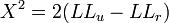$X^2 = 2 (LL_u - LL_r)$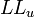$LL_u$ is the log-likelihood of the unrestricted model estimated using the Calibrated Weight,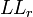$LL_r$ is the log-likelihood of the restricted model estimated using the Calibrated Weight,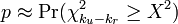$p \approx \Pr(\chi^2_{k_u - k_r} \ge X^2)$, and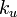$k_u$ and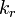$k_r$ are the number of parameters of the unrestricted and restricted models respectively.